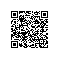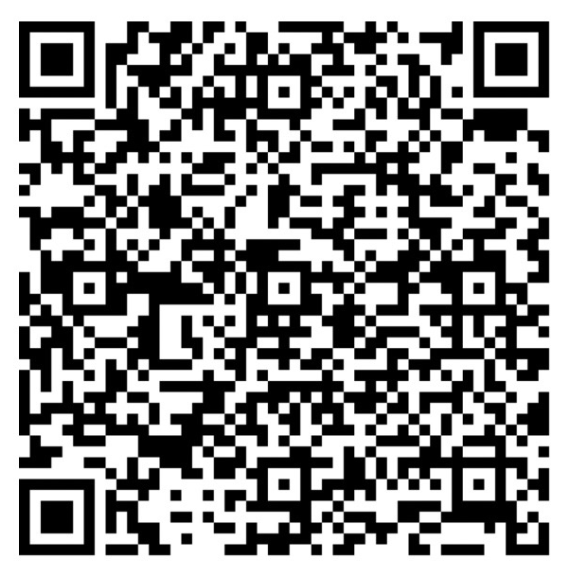# Redis实战之限制操作频率

### 场景

##### 场景1
留言功能限制，30秒 内只能评论 10次，超出次数不让能再评论，并提示：过于频繁

##### 场景2
点赞功能限制，10秒 内只能点赞 10次，超出次数后不能再点赞，并禁止操作 1个小时，提示：过于频繁，被禁止操作1小时

##### 场景3
上传记录功能，限制一天只能上传 100次，超出次数不让能再上传，并提示：超出今日上线


### 抽离本质• 限制对象：用户
• 限制操作（评论，点赞，记录， ...）
• 时间范围X秒内
• 限制操作数Y次
• 超出后禁止操作时间Z（秒/具体时间）
• 超出后不让再操作，并提示（最小时间单位用秒：天/小时/分钟都可换算成秒，用秒可以解决更多的场景）

<?php
/**
* 频率限制
* @param string $action 操作动作 * @param int$userId 发起操作的用户ID
* @param int $time 时间范围X秒内 * @param int$number 限制操作数Y次
* @param array $expire 超出封印时间Z ['type'=>1,'ttl'=>过期时间/秒] ['type'=>2,'ttl'=>具体过期时间戳] 二选一 * @return bool * @throws \Exception */ public static function frequencyLimit(string$action, int $userId, int$time, int $number,$expire = [])
{
// todo 根据用户操作动作时间范围，进行频率的控制和失效释放
}


### 解决方案落地

<?php
/**
* 频率限制
* @param string $action 操作动作 * @param int$userId 发起操作的用户ID
* @param int $time 时间范围X秒内 * @param int$number 限制操作数Y次
* @param array $expire 超出封印时间Z ['type'=>1,'ttl'=>过期时间/秒] ['type'=>2,'ttl'=>具体过期时间戳] 二选一 * @return bool * @throws \Exception */ public function frequencyLimit(string$action, int $userId, int$time, int $number,$expire = [])
{
if (empty($action) ||$userId <= 0 || $time <= 0 ||$number <= 0) {
throw new \Exception('非法参数');
}
$key = 'act:limit:' .$action . ':' . $userId;$r = RedisClient::connect();
//获取当前累计次数
$current = intval($r->get($key)); if ($current >= $number) return false; //累计并返回最新值$current = $r->incr($key);
//第一次累加，设置控制操作频率的有效时间
if ($current === 1)$r->expire($key,$time);
//未超出限制次数先放过
if ($current <$number) return true;
//超出后根据需要重新设置过期失效时间 $current ===$number 判断保证只重新设置一次
$type = empty($expire['type']) ? 0 : intval($expire['type']);$ttl = empty($expire['ttl']) ? 0 : intval($expire['ttl']);
if ($current ===$number && $ttl > 0 && in_array($type, [1, 2])) {
if ($type === 1)$r->expire($key,$ttl);
if ($type === 2)$r->expireAt($key,$ttl);
}
return false;
}
//场景1

/**
* 评论限制
* @param int $userId * @return bool|string */ public function doComment(int$userId)
{
try {
$pass = FrequencyLimit::doHandle('comment',$userId, 30, 10);
if (!$pass) return '过于频繁'; // todo 评论逻辑 return true; } catch (\Exception$e) {
return $e->getMessage(); } } //场景2 /** * 点赞限制 * @param int$userId
* @return bool|string
*/
public function doLike(int $userId) { try {$pass = FrequencyLimit::doHandle('like', $userId, 10, 10, ['type' => 1, 'ttl' => 1 * 60 * 60]); if (!$pass) return '过于频繁，被禁止操作1小时';
// todo 点赞逻辑
return true;
} catch (\Exception $e) { return$e->getMessage();
}
}

//场景3

/**
* 上传限制
* @param int $userId * @return bool|string */ public function doUpload(int$userId)
{
try {
$expire = strtotime(date('Y-m-d', strtotime(+1 . 'days')));$pass = FrequencyLimit::doHandle('upload', $userId, 1 * 24 * 60 * 60, 100, ['type' => 2, 'ttl' =>$expire]);
if (!$pass) return '超出今日上线'; // todo 上传逻辑 return true; } catch (\Exception$e) {
return \$e->getMessage();
}
}

//场景N

### 总结

• 对相似的业务场景进行分析，发现本质问题并设计通用的解决方案
• 让解决方案更有价值，做一个有灵魂的开发者
• 熟练掌握redis，充分利用它的特性和优势使用钉钉扫一扫加入圈子
+ 订阅